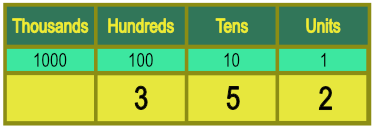Place Value

# Place Value

Basic,

We use the digits 0, 1, 2, 3, 4, 5, 6, 7, 8 and 9 to make numbers.

Where a number consists of more than one digit, the position of each digit indicates what that digit is worth. This is the place value of the digit.In the number 352, the 3 is in the Hundreds column, and is worth 3 x 100 = 300.

The 5 is in the Tens column and is worth 5 x 10 = 50.

The 2 in the Units column is worth 2.

The whole number is worth 300 + 50 + 2 = 352.

## Examples

1. What is the value of the digit 7 in the number 3706?